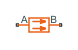# Conductive Heat Transfer

Heat transfer by conduction

•Libraries:
Simscape / Foundation Library / Thermal / Thermal Elements

## Description

The Conductive Heat Transfer block represents heat transfer by conduction between two layers of the same material. For a flat surface, the Fourier law describes the transfer,

`$Q=k\cdot \frac{A}{D}\left({T}_{A}-{T}_{B}\right),$`

where:

• Q is the heat flow.

• k is the thermal conductivity of the material.

• A is the area normal to the heat flow direction.

• D is the distance between layers, that is, the thickness of material.

• TA is the temperature of layer A.

• TB is the temperature of layer B.

Heat conduction through a round pipe wall is

`${Q}_{cyl}=2\pi k\cdot \frac{L}{\mathrm{ln}\left(\frac{{d}_{out}}{{d}_{in}}\right)}\left({T}_{A}-{T}_{B}\right),$`

where:

• L is the pipe length.

• din is the inner diameter.

• dout is the outer diameter.

You can specify the thermal conductivity by using the Conductivity type parameter:

• `Constant` — Thermal conductivity remains constant during simulation. You specify the thermal conductivity by using the Thermal conductivity parameter.

• `Variable input` — You specify the thermal conductivity using the input physical signal at port K, which can vary during simulation. The Minimum thermal conductivity parameter specifies the lower bound for the physical signal.

• `Tabulated data` — You specify the thermal conductivity by using a lookup table based on temperature. In this case, thermal conductivity can also vary during simulation.

The `Tabulated data` option uses the average temperature of the block to find the thermal conductivity. For planar wall geometry, the average temperature is

`${T}_{avg}=\frac{{T}_{A}+{T}_{B}}{2}.$`

For cylindrical wall geometry, the average temperature is

`${T}_{avg}=\left({T}_{A}-{T}_{B}\right)\cdot \left(\frac{{d}_{out}}{{d}_{out}-{d}_{in}}-\frac{1}{\mathrm{ln}\left(\frac{{d}_{out}}{{d}_{in}}\right)}\right)+{T}_{B},$`

which assumes that TB is at the inside of the cylinder.

A and B are thermal conserving ports associated with the material layers. Because the block positive direction is from port A to port B, the heat flow is positive if it flows from A to B.

### Variables

To set the priority and initial target values for the block variables prior to simulation, use the Initial Targets section in the block dialog box or Property Inspector. For more information, see Set Priority and Initial Target for Block Variables.

Nominal values provide a way to specify the expected magnitude of a variable in a model. Using system scaling based on nominal values increases the simulation robustness. Nominal values can come from different sources, one of which is the Nominal Values section in the block dialog box or Property Inspector. For more information, see Modify Nominal Values for a Block Variable.

## Ports

### Input

expand all

Input physical signal that controls the thermal conductivity. The signal saturates when the value is outside the minimum limit specified by the Minimum thermal conductivity parameter.

#### Dependencies

To enable this port, set the Conductivity type parameter to `Variable input`.

### Conserving

expand all

Thermal conserving port associated with layer A.

Thermal conserving port associated with layer B. For cylindrical wall geometry, layer B is the inside layer.

## Parameters

expand all

Whether to specify thermal conductivity as:

• `Constant` — Thermal conductivity remains constant during simulation. You specify the thermal conductivity by using the Thermal conductivity parameter.

• `Variable input` — You specify thermal conductivity using the input physical signal at port K, which can vary during simulation. The Minimum thermal conductivity parameter specifies the lower bound for the physical signal.

• `Tabulated data` — You specify thermal conductivity by using a lookup table based on temperature. In this case, thermal conductivity can also vary during simulation.

Wall shape for heat conduction, specified as:

• `Planar` — Heat transfer is through a flat, rectangular wall. Specify the wall area and thickness.

• `Cylindrical` — Heat transfer is through a round pipe wall. Specify the pipe inner diameter, outer diameter, and length.

Area of heat transfer normal to the heat flow direction.

#### Dependencies

To enable this parameter, set Wall geometry to `Planar`.

Thickness of material, that is, the distance between layers.

#### Dependencies

To enable this parameter, set Wall geometry to `Planar`.

Inner diameter of the pipe, that is, the diameter of the inner layer of material.

#### Dependencies

To enable this parameter, set Wall geometry to `Cylindrical`.

Outer diameter of the pipe, that is, the diameter of the outer layer of material.

#### Dependencies

To enable this parameter, set Wall geometry to `Cylindrical`.

Length of the pipe.

#### Dependencies

To enable this parameter, set Wall geometry to `Cylindrical`.

Thermal conductivity of the material.

#### Dependencies

To enable this parameter, set Conductivity type to `Constant`.

Lower bound for the thermal conductivity value. The input signal at port K saturates at this value to prevent the thermal conductivity from further decreasing.

#### Dependencies

To enable this parameter, set Conductivity type to `Variable input`.

Vector of thermal conductivity values that correspond to the Temperature vector parameter values. The vector size must be the same as the Temperature vector parameter. The block performs one-dimensional table lookup of thermal conductivity by using the average temperature of the block, linear interpolation, and nearest extrapolation. For more information on the interpolation and extrapolation algorithms, see PS Lookup Table (1D).

#### Dependencies

To enable this parameter, set Conductivity type to `Tabulated data`.

Vector of temperature values that correspond to the Thermal conductivity vector parameter values. The vector must be strictly increasing. The values can be nonuniformly spaced. The vector must contain at least two values.

#### Dependencies

To enable this parameter, set Conductivity type to `Tabulated data`.

## Version History

Introduced in R2007b

expand all